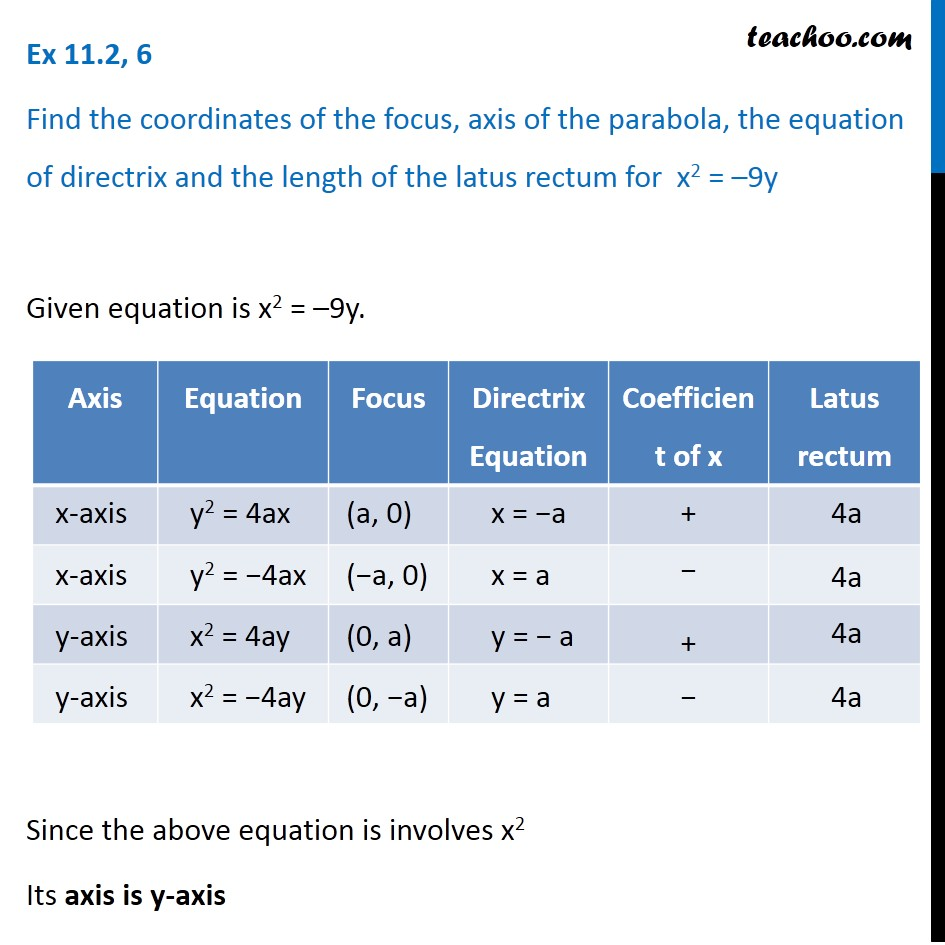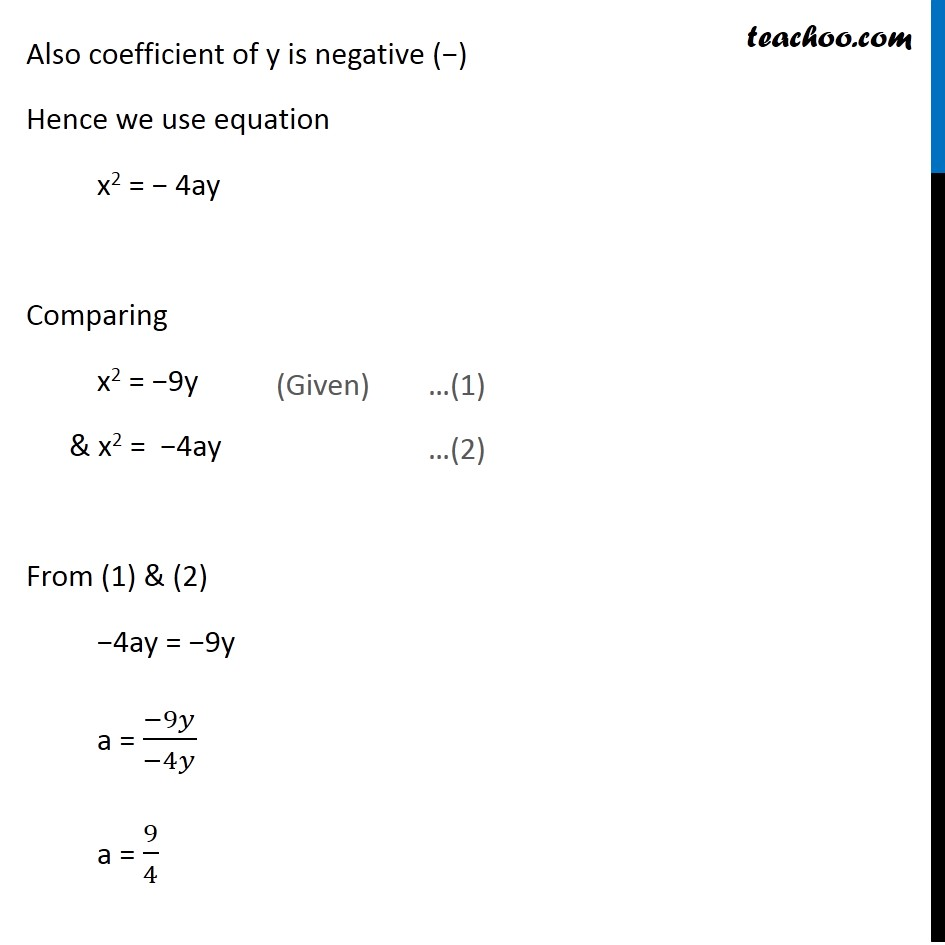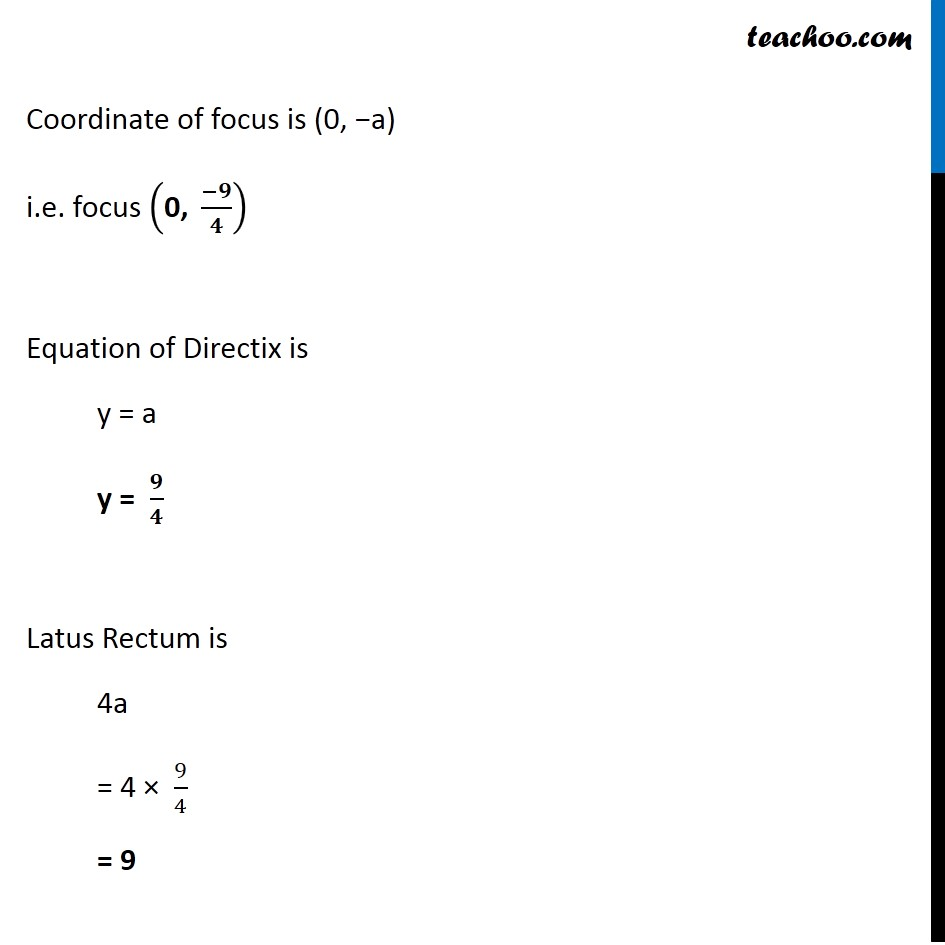Ex 10.2

Chapter 10 Class 11 Conic Sections
Serial order wiseLearn in your speed, with individual attention - Teachoo Maths 1-on-1 Class

### Transcript

Ex 10.2, 6 Find the coordinates of the focus, axis of the parabola, the equation of directrix and the length of the latus rectum for x2 = –9y Given equation is x2 = –9y. Since the above equation is involves x2 Its axis is y-axis Also coefficient of y is negative (−) Hence we use equation x2 = − 4ay Comparing x2 = −9y & x2 = −4ay From (1) & (2) −4ay = −9y a = (−9𝑦)/(−4𝑦) a = 9/4 Coordinate of focus is (0, −a) i.e. focus ("0, " (−𝟗)/𝟒) Equation of Directix is y = a y = 𝟗/𝟒 Latus Rectum is 4a = 4 × 9/4 = 9